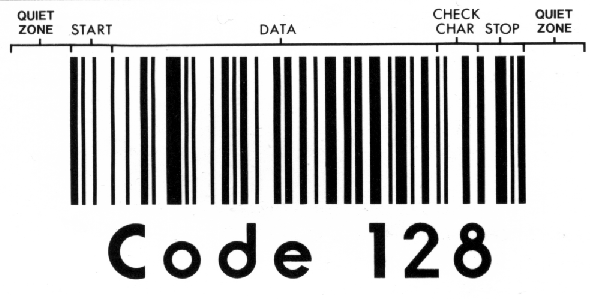## 2.6. Structure / Composition of Code 128

Table 2.2. Structure of Code 128

 one of three start codes"START "=103, or "START B"=104, or "START C"=105 the data itself a check character a stop character Termination bar of 11

We will now code the above example, HI345678, in Code 128. As we calculated in the Checksum Digit Calculation section, the checksum digit is 67. So we must also code the checksum digit at the end of the message.

We encode each digit using the encoding table above:

1. The START-A character: 11010000100
2. The digit "H" encoded as: 11000101000
3. The digit "I" encoded as: 11000100010
4. The "CODE-C" character: 10111011110
5. The digits "34" encoded as: 10001011000
6. The digits "56" encoded as: 11100010110
7. The digits "78" encoded as: 11000010100
8. The checksum digit of 67 encoded as: 10000101100
9. The STOP character: 11000111010
10. The termination bar: 11

This is shown in the following graphical representation where the bar code has been sectioned-off into areas that reflect each of the 10 components just mentioned.

Figure 2.1. Structure of Code Example for HI345678Note

Also others describe the structure Code 128 like this:

Table 2.3. Structure of Code 128 with quiet zone

 a leading quiet zone one of three start codes"START "=103, or "START B"=104, or "START C"=105 the data itself a check character a stop character a trailing quiet zone

Then this picture illuminates it well:

Figure 2.2. Structure of Code 128 with quiet zone